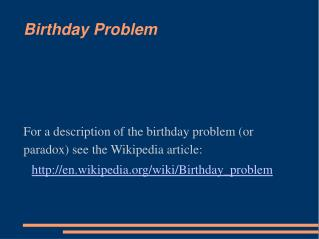DownloadDownload PresentationBirthday Problem

Birthday Problem

Télécharger la présentationBirthday Problem

- - - - - - - - - - - - - - - - - - - - - - - - - - - E N D - - - - - - - - - - - - - - - - - - - - - - - - - - -
Presentation Transcript

1. For a description of the birthday problem (or paradox) see the Wikipedia article: Birthday Problem http://en.wikipedia.org/wiki/Birthday_problem

2. Write a program that tests the result of the birthday problem. First write a boolean function, sameBirthday, that takes an integer n (the number of people in the group). The function randomly assigns birthdays to that number of people, and then checks if two or more people have the same birthday, and returns true if they do, and returns false if not. Exercise

3. The main function should loop through n, from 2 to 50, and for each n, run 5,000 trials of the birthday problem. For each n, print out the number of times that 2 or more people had the same birthday, and the probability that 2 or more people had the same birthday (the number of times divided by 5,000). You may assume a non-leap year (365 days). Exercise (cont'd)